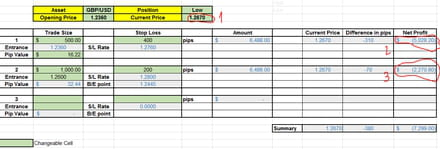# Find what is the number, that will make 2 cells ABS equal

Closed
Posts
3
Registration date
Saturday December 24, 2016
Status
Member
Last seen
December 25, 2016
-
Hey guys,

I'm trading on CFD's and trying to build something that will help me calculate different scenarios.
As you can see on the file attached, I want to build a formula in B/E point cell the price of the asset that will make two trades equal in ABS value.

In this example, I took a first trade on 1.2360. The current price is 1.2670.
pip = the change in the fourth number after the dot. for example movement from 1.2360 to 1.2357 is 3 pips movement.
Pip value on the first trade I took is 16.22\$. that means movement of 3 pips is 16.22x3=48.66\$.

second trade I took was on 1.2600, current price 1.2670. the trade is in the same direction (GBP/USD low) the pip value is 32.44\$. (higher than in the first trade. that means each pip movement in the asset price will be higher on this trade.

for example:
I'm trading LOW on GBP/USD. the price goes against me (High). moves from 1.2670 to 1.2674.
that means it moved 4 pips against me in both trades. On the first trade pip value is 16.22\$ and on the second one 32.44\$. movement of 4 pips will put me in lost of total: 16.22x4 + 32.44x4 = 194.64\$.

Now.. I want to find the Break Even point. When the lost and the profit will create 0\$ Net Profit. That meant that I want to find with a formula what is the price when the first trade and the second trade will bring to Break Even.

Questions?